# Linear Regression - an example¶

Working through a linear regression example from scratch.

Jim Mahoney | April 1 2020

### Step 0: get our software tools ready¶

In :
# dependencies
from matplotlib import pyplot as plt
from matplotlib import animation, rc
from IPython.display import HTML
import numpy as np
import random

In :
# function definitions and tests

def line(a, b, x):
""" return y value of linear model : y = a * x + b """
# This is our machine learning model :
#    · (a,b) are the parameters that define the model.
#    · x is the feature
#    · y is the target
return a * x + b

# reality check : with (a,b)=(2,3) and x=4 should be 2 * 4 + 3 = 11
assert line(2, 3, 4) == 11

def squared_length(xs):
""" return squared length of a vector (i.e. list of values) """
return sum([xs[i]**2 for i in range(len(xs)) ])

# reality check : with xs=[3,4] should be 5**2
assert squared_length([3, 4]) == 5**2

def length(xs):
return np.sqrt( squared_length(xs) )

def normalize(xs):
""" return a unit length vector in the same direction as xs """
length_xs = length(xs)
return [xs[i]/length_xs for i in range(len(xs))]

def error(a, b, xs, ys):
""" return mean sum of squared errors between ys and linear model (a,b) with features xs """
# Taking the mean makes sure that this means roughly the same thing
# independent of the size of the set we're checking, so that for example
# on a training and test set of different sizes we can compare them.
return squared_length([line(a, b, xs[i]) - ys[i] for i in range(len(xs))]) / len(xs)

def gradient_error_norm(a, b, xs, ys, da=1e-6, db=1e-6):
""" Return the normalized (i.e. length=1)
gradient (i.e. a vector rate of change in each direction = [d_error/da, d_error/db])
of the squared error length given features xs and target ys. """
# Notes :
#   * The values (da,db) are the small offsets from (a,b) used
#     to calculate the derivative numerically.
#   * Here I'm doing this by derivative by brute force differences,
#     not by taking an analytic derivative.
#   * This definition is only for this particular case,
#     with two model parameters (a,b) and a give function error().
delta_error_a = error(a+da/2, b, xs, ys) - error(a-da/2, b, xs, ys)
delta_error_b = error(a, b+db/2, xs, ys) - error(a, b-db/2, xs, ys)
return normalize( [delta_error_a / da, delta_error_b / db] )

def noise(sigma=1.0):
""" return gaussian noise value with mean=0 and given sigma"""
return random.gauss(0.0, sigma)

def scatterline(xss, yss, xlabel='x', ylabel='y',
dot_size=2, dot_color='blue', dot_label=None,
line=None, line_color='red', line_width=1.0, line_label=None,
is_first=True):
""" Draw a scatter plot (or part of a plot), which may have a line on it """
if is_first:
plt.figure(dpi=220, figsize=(2.5, 2.5))
plt.xlabel(xlabel)
plt.ylabel(ylabel)
plt.scatter(xss, yss, s=dot_size, c=dot_color, label=dot_label)
if line:
(a,b) = line
line_label = f'y = {a:0.3} x + {b:0.3}'
#plt.title(f'y = {a:0.3} x + {b:0.3}')
xs_line = [min(xss), max(xss)]
ys_line = [a * x + b for x in xs_line]
plt.plot(xs_line, ys_line, color=line_color, linewidth=line_width, label=line_label)
if dot_label or line:
plt.legend(loc='upper left', fontsize='smaller')

def indices_train_test(n, train_fraction=0.7):
""" Return list of indeces (i_train, i_test) """
i = list(range(n))                    # indices for all the data
n_train = int(n * train_fraction)     # size of training set
i_train = random.sample(i, n_train)   # random sampling without replacement
i_test = list(set(i) - set(i_train))  # indeces not in training set
return (i_train, i_test)


### Step 1 : create some data¶

I'm going to create a set of (xs_raw, ys_raw) to use that are linear with random gaussian offsets.

I'll also plot it along with the line that I used to create it, which I expect will be close to the regression line that I want to find using gradient descent.

In :
# y = a * x + b is the line I have in mind

a_raw = 1.2     # arbitrily chosen
b_raw = 3.4

n = 100         # number of points
xlow = 10       # lowest x, rest follow the line with noise() added
sigma = 15      # σ for gaussian noise (chosen by trial & error, looking at the plot)

xs_raw = [ i + noise(sigma)  for i in range(xlow, xlow + n) ]
ys_raw = [ line(a_raw, b_raw, x) + noise(sigma) for x in xs_raw ]

In :
scatterline(xs_raw, ys_raw, line=(a_raw, b_raw))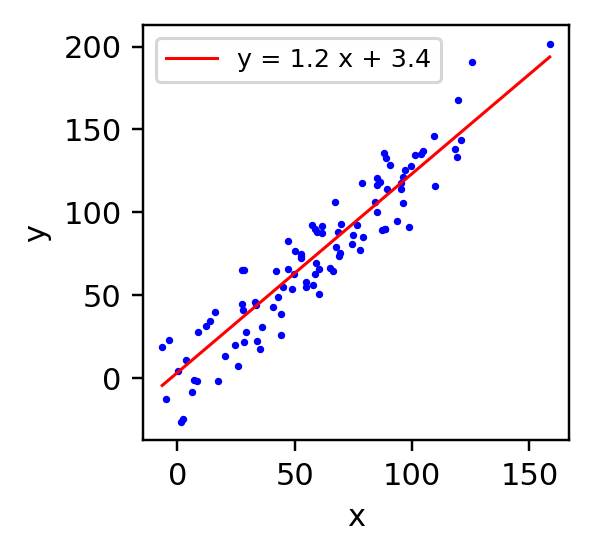### Step 2 : partition data into training and test sets¶

I'll put 70% (my default) into the training set.

And let's see what this separation looks like.

In :
(i_train, i_test) = indices_train_test(n)

xs_train = [xs_raw[i] for i in i_train]
ys_train = [ys_raw[i] for i in i_train]

xs_test = [xs_raw[i] for i in i_test]
ys_test = [ys_raw[i] for i in i_test]

In :
scatterline(xs_train, ys_train, dot_color='blue', dot_label='train')
scatterline(xs_test, ys_test, dot_color='limegreen', dot_label='test', is_first=False)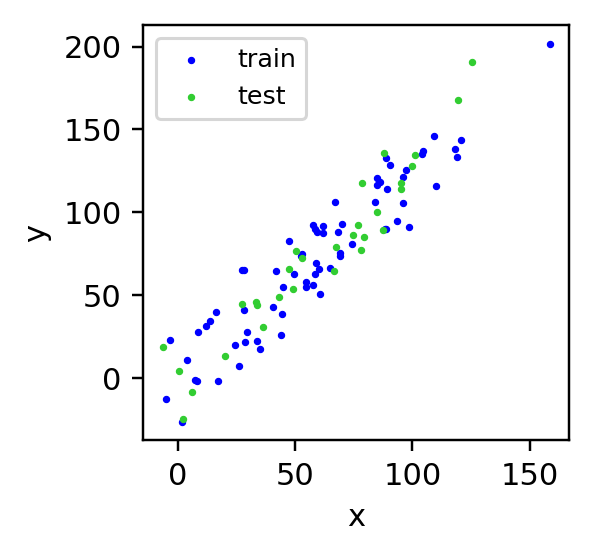### Step 3: do gradient descent to find $(a,b)$ from training data¶

Now we're ready to apply the gradient descent algorithm to numerically search for the line defined by $(a, b)$ , using the training set x_train and targets y_train to search for the minimum of the error function.

If the error function is smooth with nice minimum, like a bowl, the algorithm will decrease the error until it finds the minimum, then overshoot the best value and the error will increase.

After a bit of trial and error, I decided to decrease the step size each time the error increased, trying to converge on a better value for the lowest error.

In :
# Chose an arbitrary starting values for the linear model parameters .
(a, b) = (10.0, 20.0)    #  (line slope, line intercept)

# Choose size of each step and number of steps to take.
# TODO : stop automatically once the (a,b) converge
#        or error rate of change is very small.
step = 0.1
max_steps = 500
max_upsteps = 3
change_rate = 10000

verbose = False

# Keep track of error, a, b for each step so we can see what is happening
error_tracking = [error(a, b, xs_train, ys_train)]
a_tracking = [a]
b_tracking = [b]

# Main loop : change (a,b) in small steps in negative gradient direction
# ... until it looks like we're done.
i_step = 0
up_steps = 0
while True:
i_step += 1
(a, b) = (a + da, b + db)                               # use it to update (a, b)
change_rate = (da/a)**2 + (db/b)**2
err = error(a, b, xs_train, ys_train)
error_tracking.append(err)                              # remember err, a, b
a_tracking.append(a)                                    #  ... for later plotting
b_tracking.append(b)
d_err = error_tracking[-1] - error_tracking[-2]

if verbose:
f' a={a:6.3}  b={b:6.3}  error={err:8.4}  ' + \
f' d_err={d_err:8.5} change={change_rate:8.5}')

if d_err > 0:
up_steps += 1
step = step/2
print(f' error increased! Decreasing step size at i_step={i_step} to step={step}')

if up_steps > max_upsteps:
print(f' error increased more than {max_upsteps} times!  Stopping at i_step={i_step}')
break

if i_step > max_steps:
print(f' i_step exceeded max_steps = {max_steps}! Stopping.')
break

 error increased! Decreasing step size at i_step=91 to step=0.05
error increased! Decreasing step size at i_step=93 to step=0.025
error increased! Decreasing step size at i_step=95 to step=0.0125
error increased! Decreasing step size at i_step=98 to step=0.00625
error increased more than 3 times!  Stopping at i_step=98

In :
print("a, b = ", (a, b))

# Let's see the convergence rate :
plt.xlabel('i_step')
plt.ylabel('error')
plt.plot(range(i_step), error_tracking[:i_step]);

# And let's see if the line looks OK.
scatterline(xs_train, ys_train, line=(a, b))

a, b =  (0.9655057874598731, 19.860118680345675)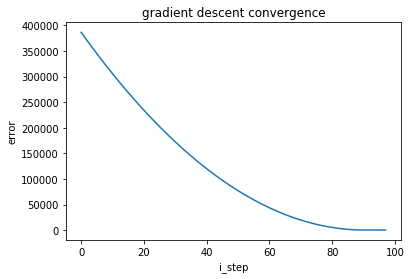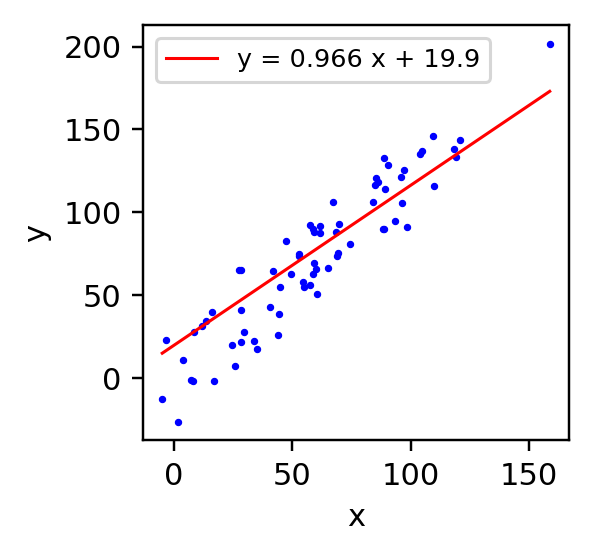Jim, can I see an animation of that?



Why not?

In :
# See http://louistiao.me/posts/notebooks/
#     embedding-matplotlib-animations-in-jupyter-as-interactive-javascript-widgets/
#
# Setting up a plot to be animated.

fig, ax = plt.subplots()
plt.scatter(xs_train, ys_train, s=2, c='blue')
plt.xlabel('x')
plt.ylabel('y')
animate_line, = ax.plot([], [], lw=2, c='red')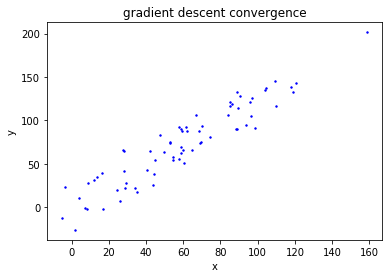In :
# Define (x,y) points for each animation step.

def init():
animate_line.set_data([], [])
return (animate_line,)

def animate(i):
y_anim = [ a_tracking[i] * x + b_tracking[i] for x in xs_train]
animate_line.set_data(xs_train, y_anim)
return(animate_line,)

anim = animation.FuncAnimation(fig, animate, init_func=init,
frames=i_step, interval=20,
blit=True)

In :
HTML(anim.to_jshtml())

Out:

Once Loop Reflect